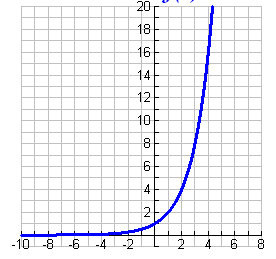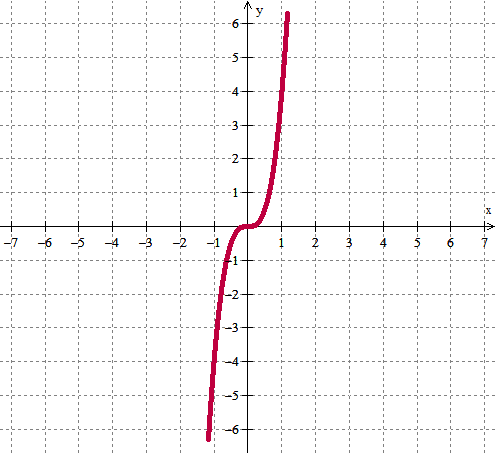MAT-HS.A-CED.02.a

 MAT-HS Targeted Standards(A) Concept: Algebra(CED) Domain: Creating ExpressionsCluster: Create equations that describe numbers or relationships MAT-HS.A-CED.02.a Create equations in two or more variables to represent relationships between quantities; graph equations on coordinate axes with labels and scales.

Student Learning Targets:

• I can
• I can

• I can
• I can

Skills (Performance) Targets

• I can create an equation in two or more variables to represent relationships between quantities.
• I can graph equations in two variables on a coordinate plane and label the axes and scales.

• I can

Proficiency Scale

 Score Description Sample Activity 4.0 In addition to achieving level 3.0 content, the student makes in-depth inferences and applications that go beyond what was taught including construct linear functions, including arithmetic and geometric sequences, given a graph, a description of a relationship, or two input-output pairs (A.F.LE.2) - 3.5 In addition to Score 3.0 performance, the student demonstrates in-depth inferences and applications regarding the more complex content with partial success. 3.0 The student can: create linear equations in two or more variables to represent relationships between quantities   graph equations on coordinate axes with labels and scales The student exhibits no major errors or omissions. Suppose $15 US is worth$150 Mexican pesos. What is an equation that relates US dollars x to Mexican pesos y? The cost to rent a car is $50 plus$0.25 per mile driven.  Write and graph an equation to represent the situation. 2.5 The student demonstrates no major errors or omissions regarding the simpler details and processes (Score 2.0 content) and partial knowledge of the more complex ideas and processes (Score 3.0 content). 2.0 There are no major errors or omissions regarding the simpler details and processes as the student: recognizes and recalls specific terminology, such as:  (A.SSE.1)                linear However, the student exhibits major errors or omissions regarding the more complex ideas and processes. - 1.5 The student demonstrates partial knowledge of the simpler details and processes (Score 2.0 content) but exhibits major errors or omissions regarding the more complex ideas and procedures (Score 3.0 content). 1.0 With help, a partial understanding of some of the simpler details and processes and some of the more complex ideas and processes. - 0.5 With help, the student demonstrates a partial understanding of some of the simpler details and processes (Score 2.0 content) but not the more complex ideas and processes (Score 3.0 content).

Alg II Create and Graph Quadratic Functions Proficiency Scale

 Score Description Sample Activity 4.0 (advanced) In addition to Score 3.0, the student demonstrates in-depth inferences and applications regarding more complex material that go beyond end of instruction expectations. Given two parabolic graphs, create the equations for both functions, graph them, and describe the similarities and differences. Function 1:  the turning point is (2,-3) and another random point on the graph is (4,5)   Function 2:  the turning point is (2,-3) and another random point on the graph is (5,6) 3.5 In addition to Score 3.0 performance, the student demonstrates in-depth inferences and applications regarding the more complex content with partial success. 3.0 (proficient) The student can: create quadratic equations in two variables given information about the function. The student exhibits no major errors or omissions. Given the x-intercepts of 6 and -2, write a quadratic function that would also pass through the point (1,5). 2.5 The student demonstrates no major errors or omissions regarding the simpler details and processes (Score 2.0 content) and partial knowledge of the more complex ideas and processes (Score 3.0 content). 2.0 (progressing) There are no major errors or omissions regarding the simpler details and processes as the student can: recognize and recall specific terminology such as: quadratic identify the graph and/or equation of quadratic functions two variables on a coordinate plane and label the axes and scales. However, the student exhibits major errors or omissions regarding the more complex ideas and processes. Given the graph below, write the quadratic function.1.5 The student demonstrates partial knowledge of the simpler details and processes (Score 2.0 content) but exhibits major errors or omissions regarding the more complex ideas and procedures (Score 3.0 content). 1.0 (beginning) With help, the student demonstrates a partial understanding of some of the simpler details and processes (Score 2.0 content) and some of the more complex ideas and processes (Score 3.0 content). 0.5 With help, the student demonstrates a partial understanding of some of the simpler details and processes (Score 2.0 content) but not the more complex ideas and processes (Score 3.0 content). 0.0 Even with help, the student demonstrates no understanding or skill.

Alg II Create and Graph Exponential Functions Proficiency Scale

 Score Description Sample Activity 4.0 (advanced) In addition to Score 3.0, the student demonstrates in-depth inferences and applications regarding more complex material that go beyond end of instruction expectations. Given two exponential graphs, create the equations for both functions, graph them, and describe the similarities and differences.Function 1:  contains the point (0, 4) and (1, 12)   Function 2:  contains the point (0, 4) and (1, 2) 3.5 In addition to Score 3.0 performance, the student demonstrates in-depth inferences and applications regarding the more complex content with partial success. 3.0 (proficient) The student can: create exponential equations in two variables given information about the function. The student exhibits no major errors or omissions. Given the points (0, 3) and (-1, 6), create the exponential equation. 2.5 The student demonstrates no major errors or omissions regarding the simpler details and processes (Score 2.0 content) and partial knowledge of the more complex ideas and processes (Score 3.0 content). 2.0 (progressing) There are no major errors or omissions regarding the simpler details and processes as the student can: recognize and recall specific terminology such as: exponential identify the graph and/or equation of exponential functions in two variables on a coordinate plane and label the axes and scales. However, the student exhibits major errors or omissions regarding the more complex ideas and processes. Given the graph below, write the exponential function.1.5 The student demonstrates partial knowledge of the simpler details and processes (Score 2.0 content) but exhibits major errors or omissions regarding the more complex ideas and procedures (Score 3.0 content). 1.0 (beginning) With help, the student demonstrates a partial understanding of some of the simpler details and processes (Score 2.0 content) and some of the more complex ideas and processes (Score 3.0 content). - 0.5 With help, the student demonstrates a partial understanding of some of the simpler details and processes (Score 2.0 content) but not the more complex ideas and processes (Score 3.0 content). 0.0 Even with help, the student demonstrates no understanding or skill. -

Alg II Create and Graph Polynomial Functions Proficiency Scale

 Score Description Sample Activity 4.0 (advanced) In addition to Score 3.0, the student demonstrates in-depth inferences and applications regarding more complex material that go beyond end of instruction expectations. Given two polynomial graphs, create the equations for both functions, graph them, and describe the similarities and differences. Function 1:  the zeros are  1, 2, 3, and another random point on the graph is (0,-6)   Function 2:  the zeros are 1, 2,3, and another random point on the graph is (0,18) 3.5 In addition to Score 3.0 performance, the student demonstrates in-depth inferences and applications regarding the more complex content with partial success. 3.0 (proficient) The student can: create polynomial equations in two variables on a coordinate plane and label the axes and scales. The student exhibits no major errors or omissions. Given the x-intercepts of -4, -1 and 2, write a polynomial function that would also pass through the point (0,-2). 2.5 The student demonstrates no major errors or omissions regarding the simpler details and processes (Score 2.0 content) and partial knowledge of the more complex ideas and processes (Score 3.0 content). 2.0 (progressing) There are no major errors or omissions regarding the simpler details and processes as the student can: recognize and recall specific terminology such as: polynomial identify the graph and/or equation of polynomial functions in two variables on a coordinate plane and label the axes and scales.    However, the student exhibits major errors or omissions regarding the more complex ideas and processes. Given the graph below, write the polynomial function.1.5 The student demonstrates partial knowledge of the simpler details and processes (Score 2.0 content) but exhibits major errors or omissions regarding the more complex ideas and procedures (Score 3.0 content). 1.0 (beginning) With help, the student demonstrates a partial understanding of some of the simpler details and processes (Score 2.0 content) and some of the more complex ideas and processes (Score 3.0 content). - 0.5 With help, the student demonstrates a partial understanding of some of the simpler details and processes (Score 2.0 content) but not the more complex ideas and processes (Score 3.0 content). 0.0 Even with help, the student demonstrates no understanding or skill. -

Resources

 Web Vocab coordinate plane scale